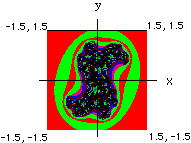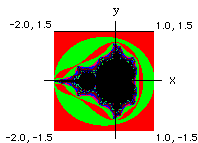Give it a try

See also the fractal explorer, for a really cool program and a far better explanation.
Also the Julia and Mandelbrot Explorer is much more comprehensive.

There are two programs available here. One is for creating Mandelbrot fractals. The other is a mistake I made while trying to create the Mandelbrot fractal generator, but still seems to generate interesting images so I left it there to play with.

• You can navigate around the plane by choosing (x, y) and then setting the field increment.
• Use the 200x200 pixel option until you find a region that looks interesting (the larger ones take more time to calculate).
• If you choose a larger pixel field, you have to modify the field increment proportionately if you want to preserve the scale.When you submit (x, y) and a field increment for x and y, the program goes over a 200 x 200 point field based on those values, calculating the behavior of each point of the field in response to (a, b). The result is a gif image with each color repesenting groups of points that behaved similarly. The starting point is the lower left corner of the image, the ending point is the upper right corner. Thus you can navigate your way around the field based on your starting point, and the size of the field increment. The corner opposite your starting point will always be (x + 200*field_inc, y + 200*field_inc) - unless you change the size of the pixel field, in which case adjust the calculation accordingly.

The program on the first form generates a field like that in the illustration. The illustration shows what the plane looks like for (a, b)=(0.35, 0.35). Starting at x, y of -1.5, -1.5 and incrementing by 0.015 in each direction over a 200X200 pixel field to end up at x, y of +1.5, +1.5. Changing the value of a and b will change the intricacy of the pattern. You can zoom in on a region by picking a point in the interesting region and adjusting the size of the field that gets plotted out.

The Mozart option is designed to work with Mozart's dice game which has been programmed by John Chuang. Mozart created a piece of music with numbered measures that could be mixed and matched based on rolling dice. The fractal generator can take the place of dice by submitting the data stream generated by the dwell set of a fractal. The dwell values are operated on by the modulus operator to give values that would be in the acceptable range of a dice roll and assignment according to Mozart's rules. The Mozart Collaborator has a good explanation of the dice game.

The program on the second form is for exploring numbers in the Mandelbrot set. A plot of the set is shown in the second illustration. You can go in and explore or amplify interesting regions by estimating the coordinates of a place with color activity, and then further navigating around with the field increment to determine how large a region you're looking at.The Mandelbrot fractal generator works based on the following:

Starting at the origin, pick a point (a, b)

Do the following iteration with the coordinates of the point:

( a*a - b*b + a , 2ab - b )
This will generate a new point (x, y)

Now go to (x, y) and ask if we are within a distance of two of the origin.

If no:

The point is not in the Mandelbrot Set, because it leaves the vicinity of the origin. Move to a new point (a + field_inrement, b + field increment) and start over.
If yes:
then plug (x, y) into the equation:
( x*x - y*y + a , 2xy - b )
Now ask if the point resulting from that is within a distance of 2 of the origin.

If the result is within a distance of 2 from the origin repeat the calculation with (a, b) on the result.
If that result is within a distance of 2 from the origin repeat the calculation with (a, b) on that result. etc. Do this iteration over and over again until the resulting point is farther than 2 from the origin, or until you feel like giving up. In this case, the program doesn't give up until it has iterated 75 times.
Numbers in the Mandelbrot set are points (a, b) that give bounded iterations. By bounded, I mean infinitely confined to the zone around the origin.
Many numbers will leave the vicinity of the origin after some number of iterations. This number is referred to as the dwell. Fractals are made by assigning a color to the point (a, b) based on its dwell. For example, numbers that remain around the origin no matter how many times they are iterated, and thus have an infinite dwell, may be assigned the color black. Points with a dwell between 1 and 5 can be red, points with dwell between 6 and 10 can be blue, etc. Once the dwell of a point has been calculated, you can move to the next point and calculate it's dwell. The trick here then becomes that question from grade school - what really is the next point? I mean there is always an infinite number of points between two points. Right? And they will all have some dwell value. Your ability to calculate it then becomes dependent on the limits of your computer hardware. You can decide the distance between points (i.e. the size of the field of points for which the dwell is calculated by setting the field increment).

Although I don't know anything about fractals, about 6 years ago I read an article in Scientific American (A.K. Dewdney, Computer Recreations, Sci. Am. February 1989) explaining various features of numbers in the "Mandelbrot set". So I wrote a little program that did what the article said. Recently in a fit of boredom I threw together the program again from memory and the result is the program above. After looking up fractals at Yahoo, I was surprised to find that there were no interactive fractal generation sites listed, so I hooked the program up to a form, and put it on my web page. In the meantime some other sites that are much more comprehensive and user friendly appeared, which I have listed above.

The program on the first form is similar to the mandelbrot fractal generator, except that (a, b) are held constant and iterated over a filed of (x, y). You pick a starting point (x, y), and a value for (a, b), and then the computer iterates with the following equation to get the dwell:

( x*x - y*y + a , 2xy - b )

with each iteration, (a, b) are held constant while (x, y) change. Once the dwell is known, the next point for iteration is (x + field_inc, y + field_inc).

Since the criteria for an interesting point is that it lie within a distance of two from the origin, it's wise to pick your starting point within 2, and also choose the field increment so that starting from your (x, y) point and moving through a 200 x 200 field of points by doing the following :

start (x, y)
calculate dwell with (a, b)

next point = ( x + field_increment, y + field_increment)
calculate dwell with (a, b)
etc.

lands you within a distance of 2 from the origin at your last point ( i.e. your last point is your first point incrememented by field_increment 200 times). Thus when the program is finished it will have mapped out the dwell on a box of 200 x 200 points in the x y plane.

Go to Fractal Generator.

Visit the User Gallery.

Here is a great site to explore more about Fractals, with a far better explanation by someone who actually knows what they're talking about.

Sorry for the shoddy explanation, I'll explain it better when I get some free time.

I'm very much indebted to Tom Boutell for being so generous as to create and provide both the gd graphics library and the cgic library, and making them both available FREE and on-line, without which creating Web stuff would be much harder. His contributions have made web creativity much more accessible.

Lastly, I realize the programs here are not very user friendly or fancy. But at the time I wrote them there was no interactive fractal stuff at Yahoo or anywhere else I looked, which was surprising. By the time this program got listed at Yahoo, other programs had sprouted up which are much more comprehensive.

Chris Seidel